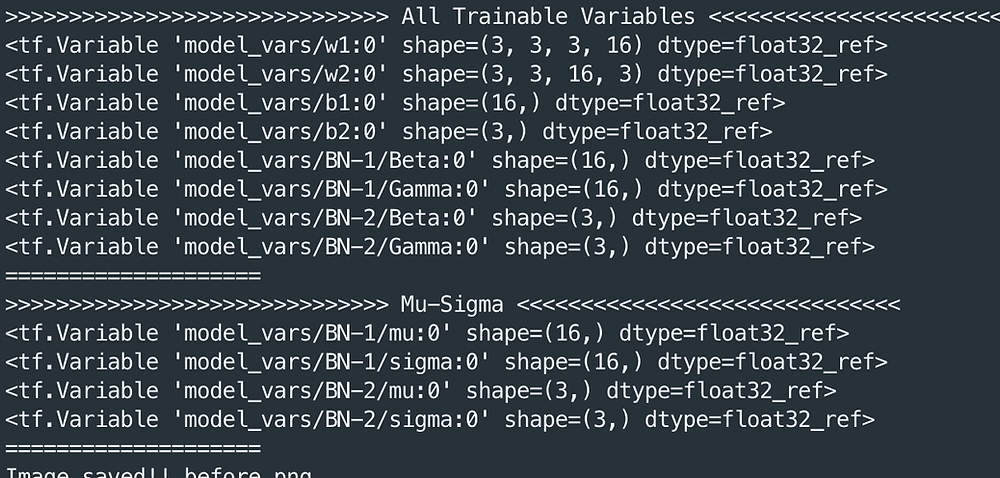Search
•Divakar V

# Batch Normalization - 1 | Using tf.nn.batch_normalization

Updated: Jul 8, 2020

The motive of this post is to share how to correctly use batch normalization in TensorFlow.

TensorFlow offers a couple of implementations for batch normalization. This post will cover tf.nn.batch_normalization api. It is a lower level api where users are themselves responsible for handling mean and variance of the input tensor.

## Background

Let's see how the Batch-Norm algorithm mentioned by the authors in the original paper (arxiv) fits into the TensorFlow tf.nn.batch_normalization api. The algorithm shown in the image is for a single mini-batch. To find mean and variance of all batches, we calculate exponentially weighted average over individual mini-batches as we progress in the training... will be covered in the code below, don't worry!Approximate mapping of original Batch Normalization algorithm (single mini-batch) to TF api
```x: Input tensor
mean: Exponential moving average over all mini-batch means. Calculated during training time.
variance: Exponential moving variance over all mini-batch variance. Calculated during training time.
offset: Beta parameter learnt by model during training.
scale: Gamma parameter learnt by model during training.
variance_epsilon: A small value (e.g. 1e-5) provided to prevent division by zero.

```

Note: The exponentially weighted mean & variance values calculated during training time, along with learnt Gamma & Beta, are used to normalize input data during test time.

I will be using a fully convolutional network to show how to use BN layer on image data. The input data will be a colored image (RGB 3-channelled). Hence the input tensor will be of the format [batch size, Height, Width, Channels]

Let's first define the parameters and the variables to be used.

Two important things to note here:

1. Batch normalization is done across [batch size, H, W]. In other words, mean & variance values are calculated over batch_size*H*W for each channel. Hence, size of variables will be number of Channels. There are other types of normalization too, such as layer-normalization, but we won't be covering it here.

2. Moving mean & moving variance variables are not to be trained by the model. So, it is important to set them as non-trainable variables. Instead, their values will be calculated as exponentially weighted averages during training time.

We have defined all the input parameters & variables to the function call:

```tf.nn.batch_normalization(
input, moving_mean, moving_variance, offset, scale, epsilon
)```

But there is an important step pending: We need to take care of moving_mean and moving_variance and update them while training the model. Remember that we set these as "non-trainable" variables. This means, they won't be updated automatically in a backward-pass during training.

This is what we need to do:

1. Calculate 1st and 2nd order moments (mean & variance) of the input tensor using tf.nn.moments().

2. Formulate updation of moving mean and variance. Better to store it as another operation variable so that you can easily call it (see below, train_mv_mean and train_mv_var).

3. Important: Now, you need to ensure that every time batch-norm layer is called, moving mean & moving variance get updated. This is important because these are non-trainable variables and won't be update in back-propagation. An easy way to do this is by adding control_dependencies. Another way could be to maintain all such (train_mv_mean and train_mv_var) variables in a list and run it separately after each epoch using sess.run().

We will be using tf.control_dependencies() as it is more convenient to use. It ensures that before running the operations defined under its scope (output_tensor), the control dependent variables (train_mv_mean, train_mv_var) run first.

During Testing time, we can simply use the moving average & moving variance of our dataset calculated during the training time. This is how the entire code looks like:

## Hands-On Code

I have written an experimental code to play around with batch normalization. It is a fully convolutional 2 layer network which takes 512 x 512 colored images (rgb 3-channeled). Output is also a 512 x 512 x 3 tensor so that it can be saved as an image easily and visualized.If you print all the trainable variables of the model, you won't find moving average and moving variance in it (as expected). You can create a separate list of these variables and print them after each epoch to see how the values are changing.Checkout out the entire code on GitHub and have fun experimenting!